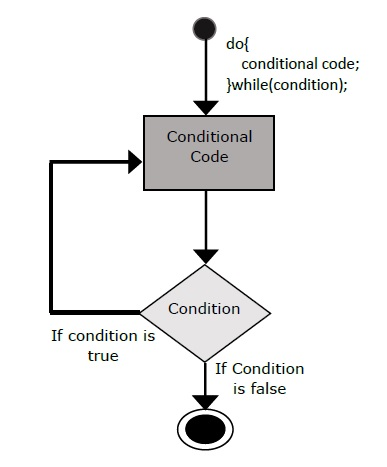# Dart Programming - do while Loop

The do…while loop is similar to the while loop except that the do...while loop doesn’t evaluate the condition for the first time the loop executes. However, the condition is evaluated for the subsequent iterations. In other words, the code block will be executed at least once in a do…while loop.

The following illustration shows the flowchart of the do…while loop −Following is the syntax for the do-while loop.

```do {
Statement(s) to be executed;
} while (expression);
```

Note − Don’t miss the semicolon used at the end of the do...while loop.

## Example

```void main() {
var n = 10;
do {
print(n);
n--;
}
while(n>=0);
}
```

The example prints numbers from 0 to 10 in the reverse order. The following output is displayed on successful execution of the above code.

```10
9
8
7
6
5
4
3
2
1
0
```
dart_programming_loops.htm